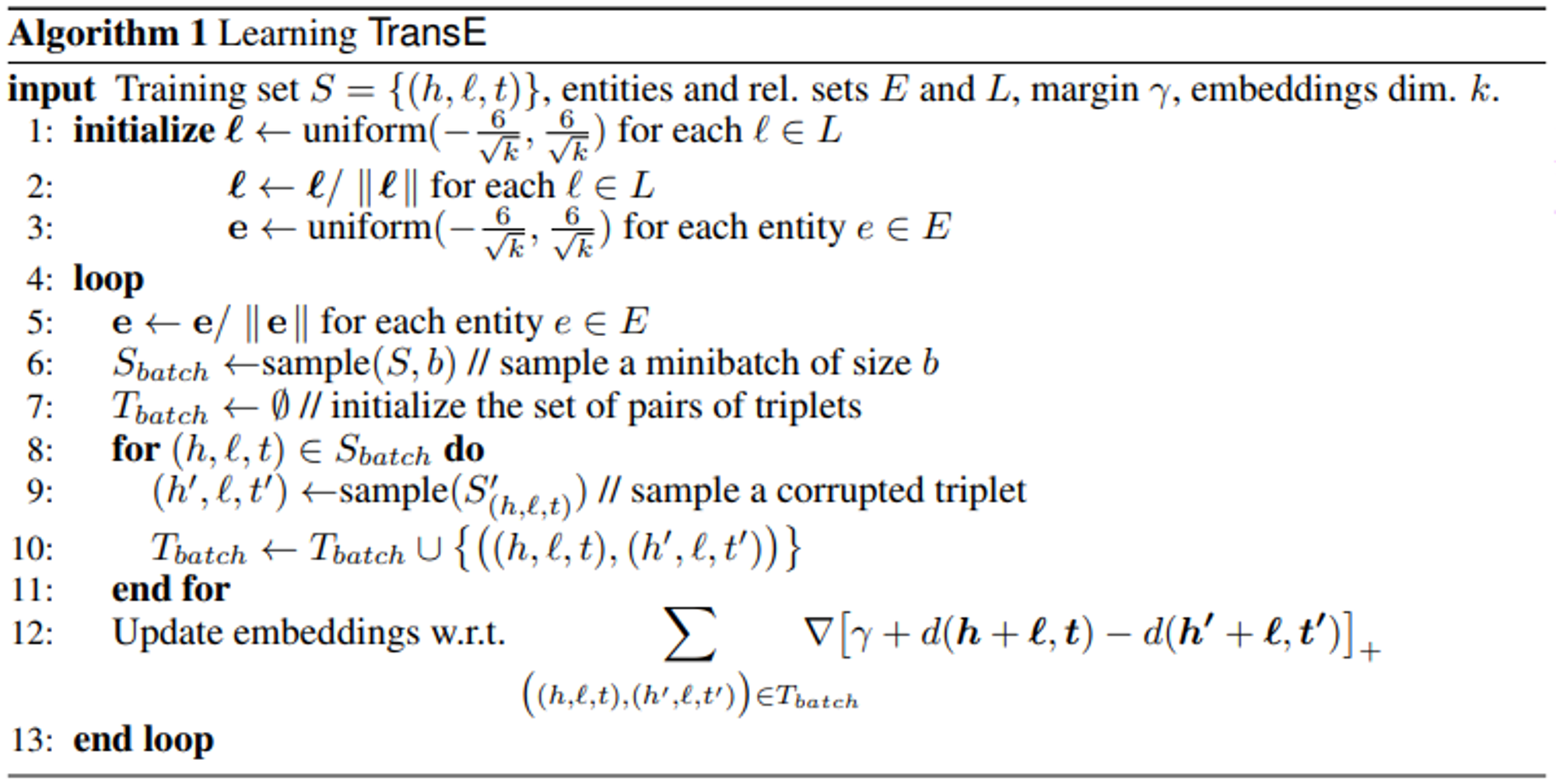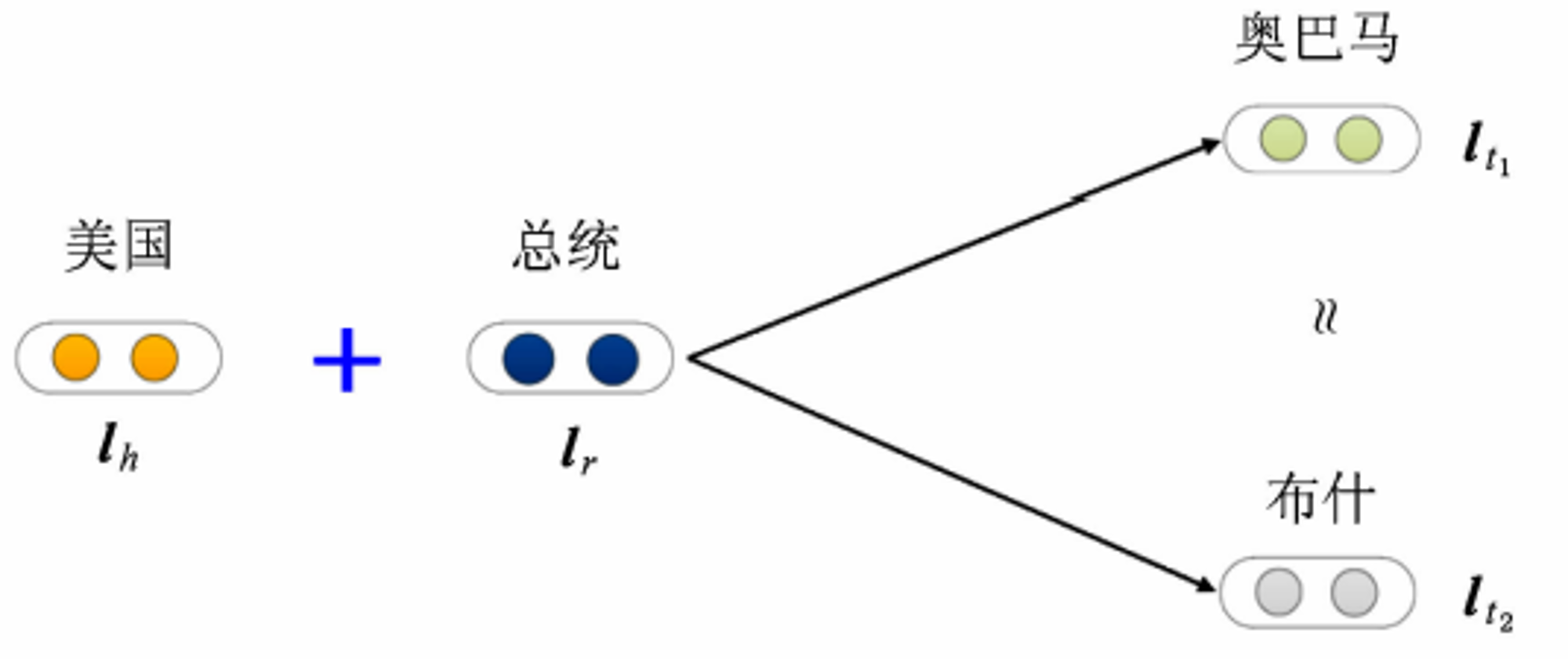V

VLyb

V1

2023/01/07阅读：57主题：默认主题

# TransE 及其实现

## 1. What is TransE?

TransE (Translating Embedding), an energy-based model for learning low-dimensional embeddings of entities.

Motivation：一是在 Knowledge Base 中，层次化的关系是非常常见的，translation 是一种很自然的用来表示它们的变换；二是近期一些从 text 中学习 word embedding 的研究发现，一些不同类型的实体之间的 1-to-1 的 relationship 可以被 model 表示为在 embedding space 中的一种 translation。

## 2. Learning TransE

TransE 的训练算法如下：### 2.1 输入参数

• training set ：用于训练的三元组的集合，entity 的集合为 ，rel. 的集合为
• margin ：损失函数中的间隔，这个在原 paper 中描述很模糊
• 每个 entity 或 rel. 的 embedding dim

### 2.2 训练过程

loop

• 在 entity embedding 被更新前进行一次归一化，这是通过人为增加 embedding 的 norm 来防止 loss 在训练过程中极小化。
• sample 出一个 mini-batch 的正样本集合
• 初始化为空集，它表示本次 loop 用于训练 model 的数据集
• for do:
• 根据 (h, l, t) 构造出一个错误的三元组
• 将 positive sample 和 negative sample 加入到
• 计算 每一对 positive sample 和 negative sample 的 loss，然后累加起来用于更新 embedding matrix。每一对的 loss 计算方式为：

loss 的计算中，

### 2.3 评价指标

1. Mean rank - 正确三元组在测试样本中的得分排名，越小越好

1. Hits@10 - 得分排名前 n 名的三元组中，正确三元组的占比，越大越好

## 3. TransE 优缺点## 4. TransE 实现

### 4.1 __init__ 函数

• ent_num：entity 的数量
• rel_num：relationship 的数量
• dim：每个 embedding vector 的维度
• norm：在计算 时是使用 L1 norm 还是 L2 norm，即
• margin：损失函数中的间隔，是个 hyper-parameter
• ：损失函数计算中的正则化项参数
class TransE(nn.Module):    def __init__(self, ent_num, rel_num, device, dim=100, norm=1, margin=2.0, alpha=0.01):        super(TransE, self).__init__()        self.ent_num = ent_num        self.rel_num = rel_num        self.device = device        self.dim = dim        self.norm = norm # 使用L1范数还是L2范数        self.margin = margin        self.alpha = alpha        # 初始化实体和关系表示向量        self.ent_embeddings = nn.Embedding(self.ent_num, self.dim)        torch.nn.init.xavier_uniform_(self.ent_embeddings.weight.data)        self.ent_embeddings.weight.data = F.normalize(self.ent_embeddings.weight.data, 2, 1)        self.rel_embeddings = nn.Embedding(self.rel_num, self.dim)        torch.nn.init.xavier_uniform_(self.rel_embeddings.weight.data)        self.rel_embeddings.weight.data = F.normalize(self.rel_embeddings.weight.data, 2, 1)        # 损失函数        self.criterion = nn.MarginRankingLoss(margin=self.margin)

torch.nn.init.xavier_uniform_ 是一个服从均匀分布的 Glorot 初始化器，在这里做的就是对 Embedding Matrix 中每个位置填充一个 xavier_uniform 初始化的值，这些值从均匀分布 中采样得到，这里的 是：

F.normalize(self.ent_embeddings.weight.data, 2, 1) 这一步就是对 ent_embeddings 的每一个值除以 dim = 1 上的 2 范数值，注意 ent_embeddings.weight.data 的 size 是 (ent_num, embs_dim)。具体来说就是这一步把每行都除以该行下所有元素平方和的开方，也就是

### 4.2 从 ent_idx 到 ent_embs

class TransE(nn.Module): ... def get_ent_resps(self, ent_idx): #[batch]        return self.ent_embeddings(ent_idx) # [batch, emb]

### 4.3 计算 energy

class TransE(nn.Module): ... def distance(self, h_idx, r_idx, t_idx):        h_embs = self.ent_embeddings(h_idx) # [batch, emb]        r_embs = self.rel_embeddings(r_idx) # [batch, emb]        t_embs = self.ent_embeddings(t_idx) # [batch, emb]        scores = h_embs + r_embs - t_embs    # norm 是计算 loss 时的正则化项        norms = (torch.mean(h_embs.norm(p=self.norm, dim=1) - 1.0)                 + torch.mean(r_embs ** 2) +                 torch.mean(t_embs.norm(p=self.norm, dim=1) - 1.0)) / 3        return scores.norm(p=self.norm, dim=1), norms

### 4.4 计算 loss

self.criterion 是通过实例化 MarginRankingLoss 得到的，这个类的初始化接收 margin 参数，实例化得到 self.criterion，其计算方式如下：

class TransE(nn.Module): ... def loss(self, positive_distances, negative_distances):        target = torch.tensor([-1], dtype=torch.float, device=self.device)        return self.criterion(positive_distances, negative_distances, target)

positive_distances 就是 ，negative_distances 就是 ，target = [-1]，代入 criterion 的计算公式就是我们计算 一对正样本和负样本的 loss 了。

### 4.5 forward

class TransE(nn.Module): ... def forward(self, ph_idx, pr_idx, pt_idx, nh_idx, nr_idx, nt_idx):        pos_distances, pos_norms = self.scoring(ph_idx, pr_idx, pt_idx)        neg_distances, neg_norms = self.scoring(nh_idx, nr_idx, nt_idx)        tmp_loss = self.loss(pos_distances, neg_distances)        tmp_loss += self.alpha * pos_norms   # 正则化项        tmp_loss += self.alpha * neg_norms   # 正则化项        return tmp_loss, pos_distances, neg_distances

V
V1